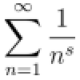Ngô Quốc Anh

October 18, 2010

1/infinity = 0 is equivalent to 1/0=infinity?

Filed under: Các Bài Tập Nhỏ, Linh Tinh — Ngô Quốc Anh @ 12:05

It is now the time to discuss some funny thing. I just learn from GR class this morning a proof of the following statement$\displaystyle \frac{1}{\infty}=0 \quad \Longleftrightarrow \quad \frac{1}{0}=\infty$.

Okay, let us start with the left hand side. By rotating 90 degrees counter-clockwise both sides of$\displaystyle \frac{1}{\infty}=0$

we get$\displaystyle -18=0$.

Now adding both sides by 8 we arrive at$\displaystyle -10=8$.

Again, rotating 90 degrees clockwise both sides we reach to$\displaystyle \frac{1}{0}=\infty$.

The reverse case can be treated similarly.

1 Comment »

1.lol amazing trick!! 😀

Comment by zariski — October 18, 2010 @ 19:34

This site uses Akismet to reduce spam. Learn how your comment data is processed.